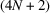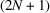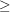International Tables for Crystallography (2006). Vol. A, ch. 10.1, pp. 762-803https://doi.org/10.1107/97809553602060000520

## Contents

• 10.1. Crystallographic and noncrystallographic point groups  (pp. 762-803)
• 10.1.1. Introduction and definitions  (p. 762) | html | pdf |
• 10.1.2. Crystallographic point groups  (pp. 763-795) | html | pdf |
• 10.1.2.1. Description of point groups  (pp. 763-764) | html | pdf |
• 10.1.2.2. Crystal and point forms  (pp. 764-766) | html | pdf |
• 10.1.2.3. Description of crystal and point forms  (p. 766) | html | pdf |
• 10.1.2.4. Notes on crystal and point forms  (pp. 766-795) | html | pdf |
• 10.1.2.5. Names and symbols of the crystal classes  (p. 795) | html | pdf |
• 10.1.3. Subgroups and supergroups of the crystallographic point groups  (pp. 795-796) | html | pdf |
• 10.1.4. Noncrystallographic point groups  (pp. 796-803) | html | pdf |
• 10.1.4.1. Description of general point groups  (pp. 796-797) | html | pdf |
• 10.1.4.2. The two icosahedral groups  (pp. 797-802) | html | pdf |
• 10.1.4.3. Sub- and supergroups of the general point groups  (pp. 802-803) | html | pdf |
• References | html | pdf |
• Figures
• Fig. 10.1.3.1. Maximal subgroups and minimal supergroups of the two-dimensional crystallographic point groups  (p. 795) | html | pdf |
• Fig. 10.1.3.2. Maximal subgroups and minimal supergroups of the three-dimensional crystallographic point groups  (p. 796) | html | pdf |
• Fig. 10.1.4.1. Subgroups and supergroups of the two-dimensional general point groups  (p. 802) | html | pdf |
• Fig. 10.1.4.2. The subgroups of the two-dimensional general point groups 16 mm (4 N -gonal system) and 18 mm [-gonal system, including the-gonal groups]  (p. 802) | html | pdf |
• Fig. 10.1.4.3. Subgroups and supergroups of the three-dimensional general point groups  (p. 803) | html | pdf |
• Tables
• Table 10.1.1.1. The ten two-dimensional crystallographic point groups, arranged according to crystal system  (p. 762) | html | pdf |
• Table 10.1.1.2. The 32 three-dimensional crystallographic point groups, arranged according to crystal system ( cf. Chapter 2.1)  (p. 763) | html | pdf |
• Table 10.1.2.1. The ten two-dimensional crystallographic point groups  (pp. 768-769) | html | pdf |
• Table 10.1.2.2. The 32 three-dimensional crystallographic point groups  (pp. 770-790) | html | pdf |
• Table 10.1.2.3. The 47 crystallographic face and point forms, their names, eigensymmetries, and their occurrence in the crystallographic point groups (generating point groups)  (pp. 791-793) | html | pdf |
• Table 10.1.2.4. Names and symbols of the 32 crystal classes  (p. 794) | html | pdf |
• Table 10.1.4.1. Classes of general point groups in two dimensions ( N = integer0)  (p. 798) | html | pdf |
• Table 10.1.4.2. Classes of general point groups in three dimensions ( N = integer0)  (pp. 798-799) | html | pdf |
• Table 10.1.4.3. The two icosahedral point groups  (pp. 800-801) | html | pdf |